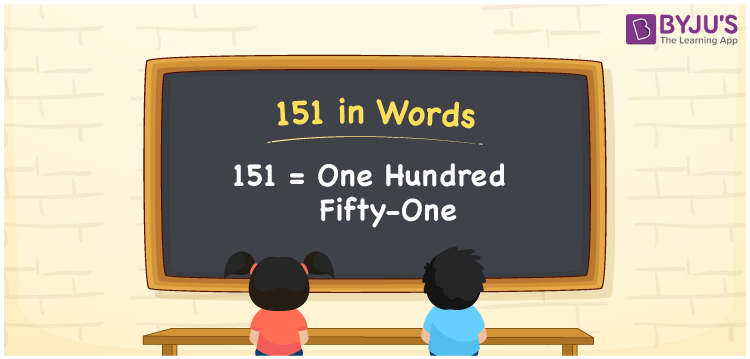# 151 in Words

151 in words is expressed as One Hundred Fifty-One. For example, if you buy a jute bag worth Rs. 151, you can say, “I bought a jute bag worth Rupees One Hundred Fifty-One”. We know that 151 is a cardinal number since it shows the value of something. Place value charts play a significant role in writing the number name with ease. Let us learn how to express the number 151 into words using a place value system in this article.

 151 in Words One Hundred Fifty-One One Hundred Fifty-One in numerical form 151

## 151 in English Words

Generally, we write numbers in words using the letters of the English alphabet to help students understand the numbers in word form in an efficient manner. Hence, in English, the number 151 can be read as One Hundred Fifty-One.## How to Write 151 in Words?

To express the place value chart for 151, let us create a table of 3 columns as 151 is a three-digit number. The below table shows the place value chart for the number 151 along with the expanded form.

 Hundreds Tens Ones 1 5 1

Therefore, we can write the expanded form as:

1 x Hundred + 5 x Ten + 1 x One

= 1 x 100 + 5 x 10 + 1 x 1

= 100 + 50 + 1

= 151

= One Hundred Fifty-One

Hence, 151 in words is written as One Hundred Fifty-One

Interesting way of writing 151 in words

1 = One

15 = Fifteen

151 = One Hundred Fifty-One

Thus, the word form of the number 151 is One Hundred Fifty-One

151 is a natural number that precedes 152 and succeeds 150

• 151 in words – One Hundred Fifty-One
• Is 151 an odd number? – Yes
• Is 151 an even number? – No
• Is 151 a perfect square number? – No
• Is 151 a perfect cube number? – No
• Is 151 a prime number? – Yes
• Is 151 a composite number? – No

## Frequently Asked Questions on 151 in Words

### How to write 151 in words?

We can write 151 in words as One Hundred Fifty-One.

### Simplify 100 + 51, and express in words.

Simplifying 100 + 51, we get 151. Thus, the number 151 in words is One Hundred Fifty-One.

### Convert One Hundred Fifty-One in numbers.

One Hundred Fifty-One in numbers is 151.